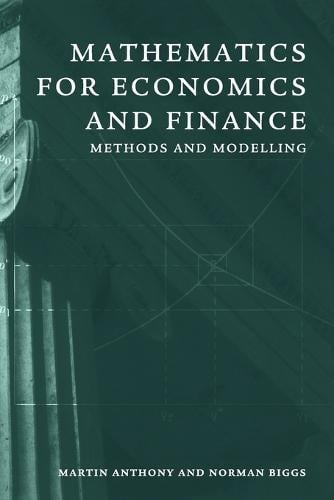•Mathematics for Economics and Finance: Methods and Modelling (Paperback)

(author), (author)
£40.99
Paperback 407 Pages / Published: 13/07/1996
• 10+ in stock
• Free UK delivery

Available

Your local Waterstones may have stock of this item. Please check by using Click & Collect
Mathematics has become indispensable in the modelling of economics, finance, business and management. Without expecting any particular background of the reader, this book covers the following mathematical topics, with frequent reference to applications in economics and finance: functions, graphs and equations, recurrences (difference equations), differentiation, exponentials and logarithms, optimisation, partial differentiation, optimisation in several variables, vectors and matrices, linear equations, Lagrange multipliers, integration, first-order and second-order differential equations. The stress is on the relation of maths to economics, and this is illustrated with copious examples and exercises to foster depth of understanding. Each chapter has three parts: the main text, a section of further worked examples and a summary of the chapter together with a selection of problems for the reader to attempt. For students of economics, mathematics, or both, this book provides an introduction to mathematical methods in economics and finance that will be welcomed for its clarity and breadth.

Publisher: Cambridge University Press
ISBN: 9780521559133
Number of pages: 407
Weight: 658 g
Dimensions: 228 x 152 x 23 mm

MEDIA REVIEWS
'Throughout, the stress is firmly on how the mathematics relates to economics, and this is illustrated with copious examples and exercises that will foster depth of understanding.' L'Enseignement Mathematique